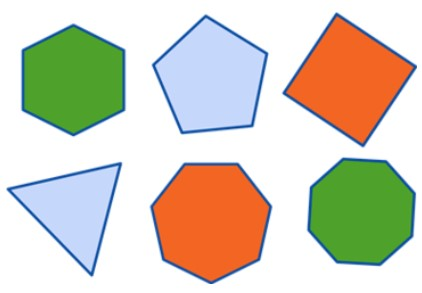# Polygon Calculator

Choose from the list and enter the values of required properties to find the area of a polygon using the area of a polygon with apothem calculator.

Give Us Feedback

Polygon area calculator finds the area of a polygon using different sets of values i.e length and the number of sides or radius (apothem) and the number of sides.

The fun fact is, the results include perimeter and area in both ways:

• Using length

## What is a Polygon?

Polygon refers to a plane geometrical figure or shape that has at least three or more sides and angles.A polygon with three sides is called a triangle, A polygon with four sides is called a square e.t.c

## Area of Polygon formula

The formula of a polygon using length sides:

Area = a2.n/ 4tan (π/n)

Where,

a = length of side

n = Number of sides

The formula of a polygon using inradius or apothem.

Area = n x ri x tan(π/n) / 2

Where,

n = Number of sides